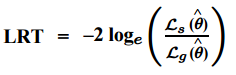# Likelihood-Ratio Tests (Probability and Mathematical Statistics)

Hypothesis Testing Likelihood-Ratio Tests

## What is a Likelihood-Ratio Test?

The Likelihood-Ratio test (sometimes called the likelihood-ratio chi-squared test) is a hypothesis test that helps you choose the “best” model between two nested models. “Nested models” means that one is a special case of the other. For example, you might want to find out which of the following models is the best fit:

• Model One has four predictor variables (height, weight, age, sex),
• Model Two has two predictor variables (age,sex). It is “nested” within model one because it has just two of the predictor variables (age, sex).

This theory cam also be applied to matrices. For example, a scaled identity matrix is nested within a more complex compound symmetry matrix.

The best model is the one that makes the data most likely, or maximizes the likelihood function“>likelihood function, fn(X – 1, … , Xn|Θ).
Although the concept is relatively easy to grasp (i.e. the likelihood function is highest nearer the true value for Θ), the calculations to find the inputs for the procedure are not.

Likelihood-ratio tests use log-likelihood functions, which are difficult and lengthy to calculate by hand. Most statistical software packages have built in functions to handle them; On the other hand, log-likelihood functions pose other serious challenges, like the difficulty of calculating global maximums. These often involve hefty computations with complicated, multi-dimensional integrals.

## Running the Test

Basically, the test compares the fit of two models. The null hypothesis is that the smaller model is the “best” model; It is rejected when the test statistic is large. In other words, if the null hypothesis is rejected, then the larger model is a significant improvement over the smaller one.

If you know the log-likelihood functions for the two models, the test statistic is relatively easy to calculate as the ratio between the log-likelihood of the simpler model (s) to the model with more parameters (g):You might also see this equation with “s” written as the likelihood for the null model and “g” written as the likelihood for the alternative model.

The test statistic approximates a chi-squared random variable. Degrees of freedom for the test equal the difference in the number of parameters for the two models.

CITE THIS AS:
Stephanie Glen. "Likelihood-Ratio Tests (Probability and Mathematical Statistics)" From StatisticsHowTo.com: Elementary Statistics for the rest of us! https://www.statisticshowto.com/likelihood-ratio-tests/